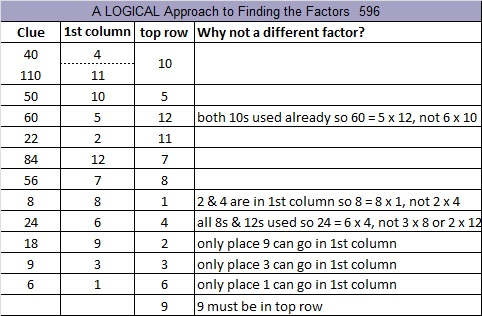596 Select the Correct Top Row

596 is the sum of the four primes numbers from 139 to 157. The two prime numbers in the middle of that sum are twin primes. Do you know what they are?

596 is also the hypotenuse of the Pythagorean triple 204-560-596. What is the greatest common factor of those three numbers?Print the puzzles or type the solution on this excel file: 12 Factors 2015-08-24

—————————————————————————————————

• 596 is a composite number.
• Prime factorization: 596 = 2 x 2 x 149, which can be written 596 = (2^2) x 149
• The exponents in the prime factorization are 1 and 2. Adding one to each and multiplying we get (1 + 1)(2 + 1) = 2 x 3  = 6. Therefore 596 has exactly 6 factors.
• Factors of 596: 1, 2, 4, 149, 298, 596
• Factor pairs: 596 = 1 x 596, 2 x 298, or 4 x 149
• Taking the factor pair with the largest square number factor, we get √596 = (√4)(√149) = 2√149 ≈ 24.41311123—————————————————————————————————